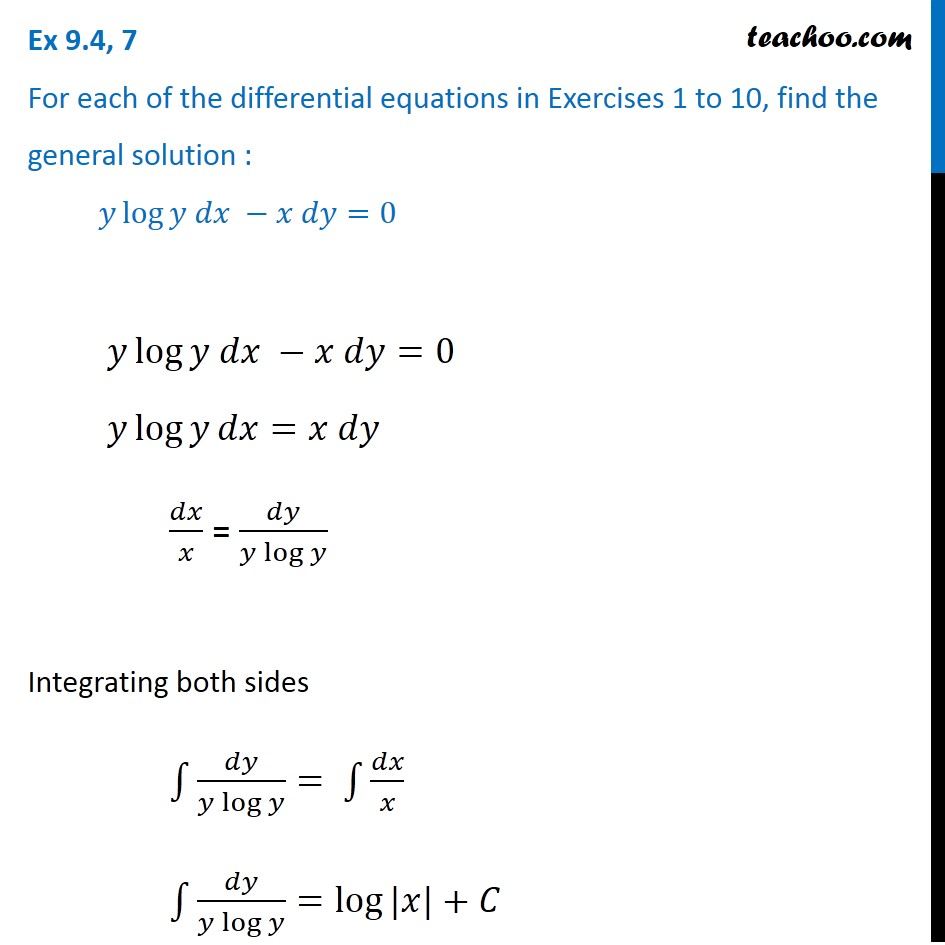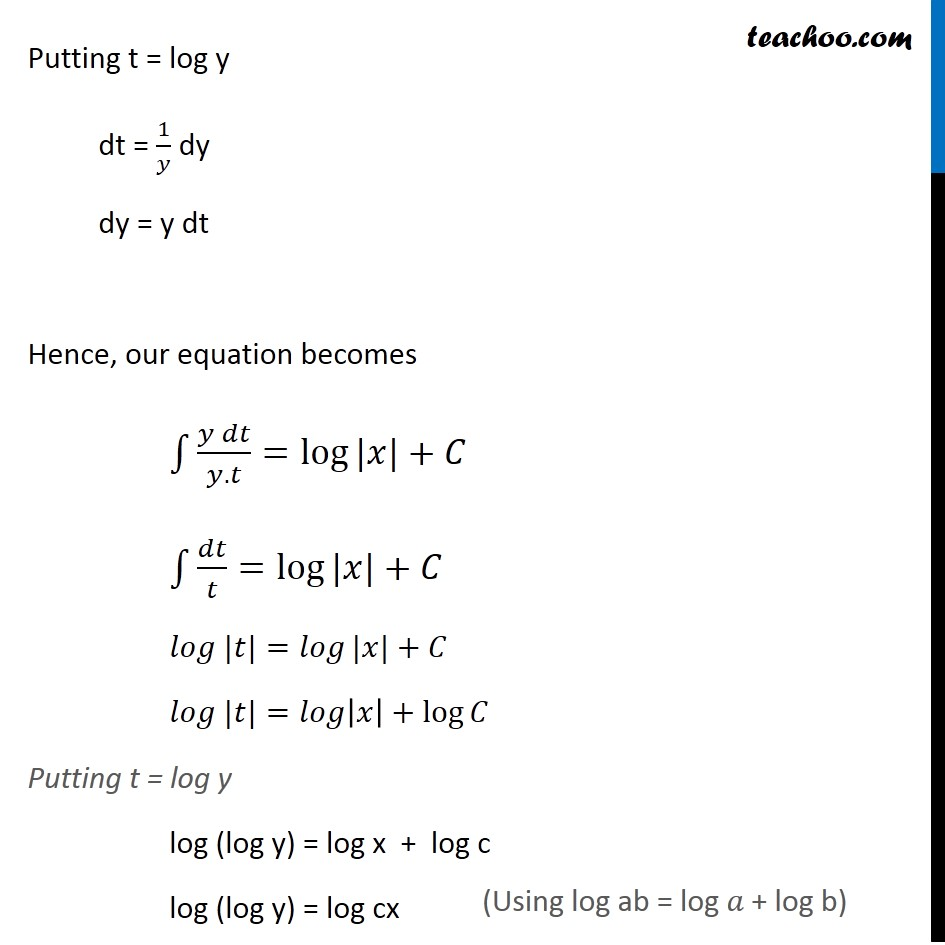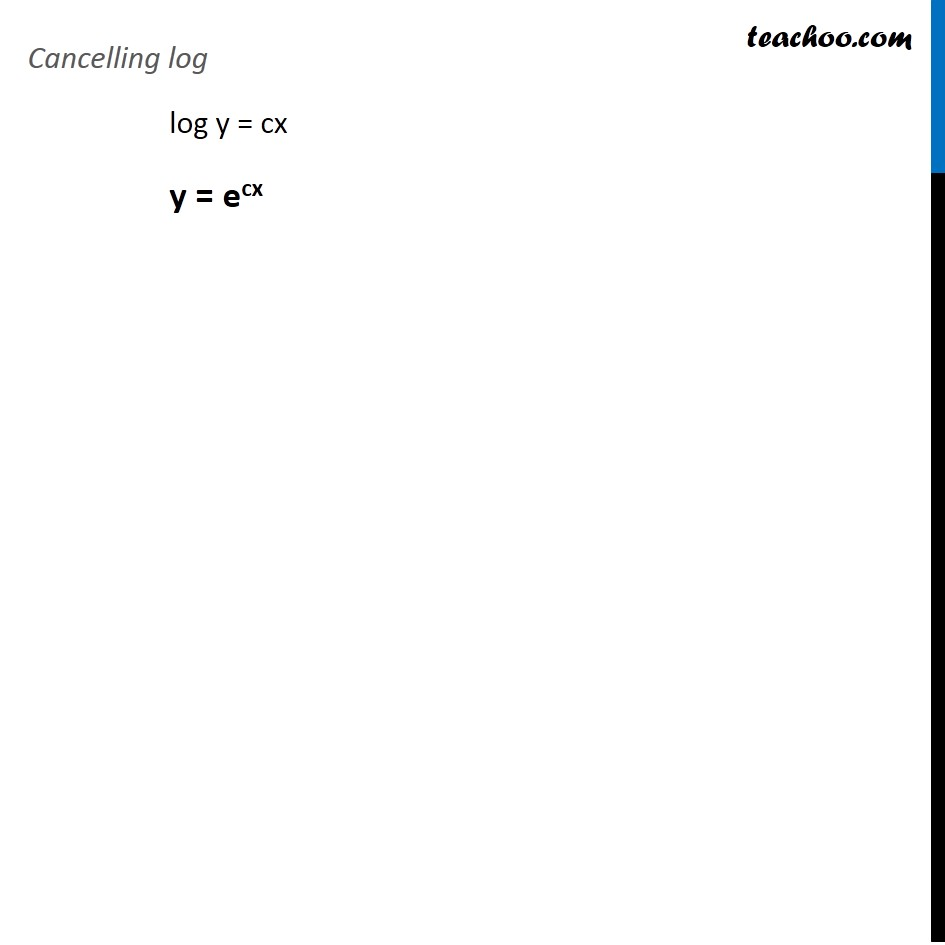Ex 9.3

Chapter 9 Class 12 Differential Equations
Serial order wiseLearn in your speed, with individual attention - Teachoo Maths 1-on-1 Class

### Transcript

Ex 9.3, 7 For each of the differential equations in Exercises 1 to 10, find the general solution : 𝑦 log⁡〖𝑦 𝑑𝑥 −𝑥 𝑑𝑦=0〗 𝑦 log⁡〖𝑦 𝑑𝑥 −𝑥 𝑑𝑦=0〗 𝑦 log⁡𝑦 𝑑𝑥=𝑥 𝑑𝑦 𝑑𝑥/𝑥 = 𝑑𝑦/(𝑦 log⁡𝑦 ) Integrating both sides ∫1▒〖𝑑𝑦/(𝑦 log⁡𝑦 )= ∫1▒𝑑𝑥/𝑥〗 ∫1▒𝑑𝑦/(𝑦 log⁡𝑦 )=log⁡〖|𝑥|〗+𝐶 Putting t = log y dt = 1/𝑦 dy dy = y dt Hence, our equation becomes ∫1▒(𝑦 𝑑𝑡)/(𝑦.𝑡)=log⁡〖|𝑥|〗+𝐶 ∫1▒𝑑𝑡/𝑡=log⁡〖|𝑥|〗+𝐶 𝑙𝑜𝑔 |𝑡|=𝑙𝑜𝑔⁡〖|𝑥|〗+𝐶 𝑙𝑜𝑔 |𝑡|=𝑙𝑜𝑔⁡|𝑥|+log⁡𝐶 Putting t = log y log (log y) = log x + log c log (log y) = log cx (Using log ab = log 𝑎 + log b) Cancelling log log y = cx y = ecx